Next: Equation of Time Up: The Sun Previous: Example Longitude Calculations

Determination of Equinox and Solstice Dates

We can also use Tables 32 and 33 to calculate the dates of the equinoxes and solstices, and, hence, the lengths of the seasons, in a given year. The vernal equinox (i.e., the point on the sun's apparent orbit at which it passes through the celestial equator from south to north) corresponds to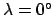, the summer solstice (i.e., the point at which the sun is furthest north of the celestial equator) to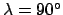, the autumnal equinox (i.e., the point at which the sun passes through the celestial equator from north to south) to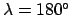, and the winter solstice (i.e., the point at which the sun is furthest south of the celestial equator) to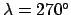--see Fig. 22. Furthermore, spring is defined as the period between the spring equinox and the summer solstice, summer as the period between the summer solstice and the autumnal equinox, autumn as the period between the autumnal equinox and the winter solstice, and winter as the period between the winter solstice and the following vernal equinox. Consider the year 2000 CE. For the case of the vernal equinox, we can first estimate the time at which this event takes place by approximating the solar longitude as the mean solar longitude: i.e.,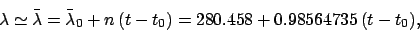We obtain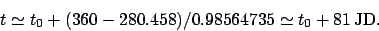Calculating the true solar longitude at this time, using Tables 32 and 33, we get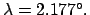Now, the actual vernal equinox occurs when. Thus, a much better estimate for the date of the vernal equinox is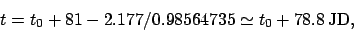which corresponds to 7:00 UT on March 20. Similar calculations show that the summer solstice takes place at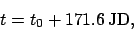corresponding to 2:00 UT on June 21, that the autumnal equinox takes place at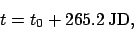corresponding to 17:00 UT on September 22, and that the winter solstice takes place at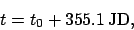corresponding to 14:00 UT on December 21. Thus, the length of spring is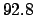days, the length of summer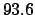days, and the length of autumn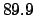days. Finally, the length of winter is the length of the tropical year (i.e., the time period between successive vernal equinoxes), which is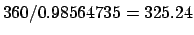days, minus the sum of the lengths of the other three seasons. This gives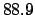days.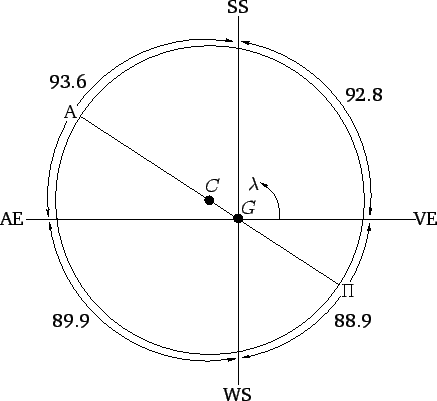Figure 22 illustrates the relationship between the equinox and solstice points, and the lengths of the seasons. The earth is displaced from the geometric center of the sun's apparent orbit in the direction of the solar perigee, which presently lies between the winter solstice and the vernal equinox. This displacement (which is greatly exaggerated in the figure) has two effects. Firstly, it causes the arc of the sun's apparent orbit between the summer solstice and autumnal equinox to be longer than that between the winter solstice and the vernal equinox. Secondly, it causes the sun to appear to move faster in winter than in summer, in accordance with Kepler's second law, since the sun is closer to the earth in the former season. Both of these effects tend to lengthen summer, and shorten winter. Hence, summer is presently the longest season, and winter the shortest.Next: Equation of Time Up: The Sun Previous: Example Longitude Calculations
Richard Fitzpatrick 2010-07-21